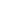Flat 50% off

Ends in

• JEE Main
•• 11th
•• Physics
• Back to Doubt Clearing

## Hello Sir please solve this question : The coordinates of a moving particle at any time $t$ are given by $x = \alpha t^3$ and $y = \beta t^3$. The speed of the particle at time $t$ is given by (a) $t^2 \sqrt {\alpha + \beta}$ (b) $t^3 \sqrt {\alpha^3 + \beta^2}$ (c) $3t^2 \sqrt {\alpha ^2+ \beta^2}$ (d) $3t \sqrt {\alpha ^2+ \beta^2}$

### Asked By Vimal

Updated Fri, 10 Aug 2018 02:16 pm